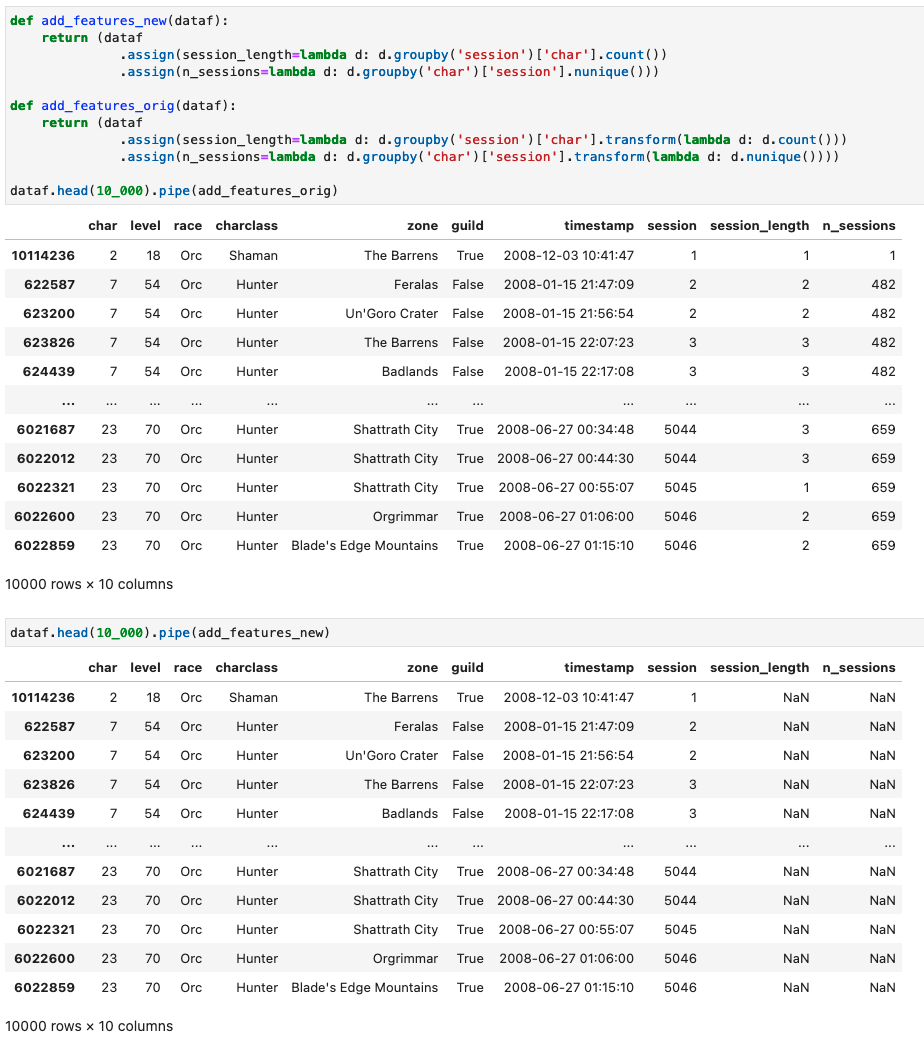Instantly share code, notes, and snippets.

# koaning/polars.ipynb

Created November 1, 2021 20:54
Star You must be signed in to star a gist
A benchmark with Polars.
Display the source blob
Display the rendered blob
Raw
Sorry, we cannot display this file.
Sorry, this file is invalid so it cannot be displayed.

### wgong commented Feb 18, 2023 • edited

Thank you for a great tutorial on polars - https://calmcode.io/polars/introduction.html

On my HP laptop (AMD Ryzen 5 2.10 GHz 16 GB Ram),
I see a much bigger speedup by 137

``````polars (0.15.14) 0m 3.84s
pandas (v1.4.4) 8m 47s
``````

Go Polars

### dshemetov commented Mar 26, 2023

While I am a fan of Calmcode and accept that Polars is faster in many cases, I think this benchmark is selling Pandas short. The main reason the Pandas code is slow is because it's using `GroupBy.transform`, which is actually slow Python for-loop over the groups under the hood. Changing those functions to use the Pandas built-ins (like `GroupBy.count` or `GroupBy.nunique`), I get the benchmarks

``````polars (0.16.16) 0m 4.5s
pandas (1.5.3) 0m 6.5s
``````
```def add_features(dataf):
return (dataf
.assign(session_length=lambda d: d.groupby('session')['char'].count())
.assign(n_sessions=lambda d: d.groupby('char')['session'].nunique()))

def remove_bots(dataf, max_session_hours=24):
n_rows = max_session_hours*6
return (dataf
.assign(max_sess_len=lambda d: d.groupby('char')['session_length'].max())
.loc[lambda d: d["max_sess_len"] < n_rows]
.drop(columns=["max_sess_len"]))```

TL;DR: Avoid using `transform, apply, aggregate` in performant Pandas code!

### koaning commented Mar 27, 2023

This is a fair comment! I responded on the calmcode repo as well, will dive into this a bit and run a proper benchmark on my end. Something is telling me the pandas 2.0 version might also speed things up.

### koaning commented Mar 28, 2023

I'm looking at the numbers now, and while it's totally fair to nuance my numbers ... I just want to check on a few details.

The new `add_features` and `remove_bots` pandas functions run in 2.95 seconds on my machine. But! The `set_types` and `sessionnize` functions take 14.9s and reading in the .csv file takes 5.72s. That's a total of 23.57s for pandas. I also re-ran polars, which became faster in the meantime and ran in ~2.1s.

So it's certainly not a 70x speedup, but it seems like I'm still looking at a 5.72 + 14.9 + 2.95)/2.1 = 11.2x speedup. Or, have I skipped a step? Figured I'd check.

### koaning commented Mar 28, 2023 • edited

On more diving deeper, I should immediately mention that the polars runtime does fluctuate between 2s-5s. So maybe it's more like a 4x-11x speedup.

### koaning commented Mar 28, 2023 • edited

I'm also not 100% sure if your method calculates the same thing as polars. Just to check, your method:

```def add_features(dataf):
return (dataf
.assign(session_length=lambda d: d.groupby('session')['char'].count())
.assign(n_sessions=lambda d: d.groupby('char')['session'].nunique()))```

When you call `d.groupby('session')['char'].count()` you get a series back that has a different length than the original dataframe.

```dataf = df.pipe(set_types).pipe(sessionize)
dataf.groupby("session")["char"].count().shape, dataf.shape
# ((5954828,), (10826734, 8))```

This is confirmed when looking at the output of the function.Figured I'd stop here and check for feedback, it might certainly be that I'm skipping over something, and I'll gladly hear it if that is the case.

### dshemetov commented Mar 28, 2023 • edited

Ah you're right, thanks! This is a bit more tricky than I thought.

I overlooked that the `pandas.DataFrame.transform` returns a DataFrame with the same dimension as the input DataFrame, so your original code avoids having to do a join, while my first revision above misses that.

Here's another revision that uses `join` instead of `assign`. This fixes these issues and is about an order magnitude faster than the original! I added a compare method to make sure the new code and the original code have identical outputs!

I'm getting these benchmarks for the full pipeline (set types, sessionize, add features, and remove bots) in Polars and Pandas (after loading both into a df):

``````polars: 4.23 s
pandas: 23.5 s
``````

So I agree with you that Polars is almost an order of magnitude faster here, but at least it's not two orders! 😄

(Also minor note, not important for the benchmark: the sessionize default thresholds are set differently for Polars vs Pandas.)

```import pandas as pd

%%time
pandas_df.columns = [c.replace(" ", "") for c in pandas_df.columns]
# CPU times: user 5.8 s, sys: 1.21 s, total: 7 s

def set_types(dataf):
return (dataf
.assign(timestamp=lambda d: pd.to_datetime(d['timestamp'], format="%m/%d/%y %H:%M:%S"),
guild=lambda d: d['guild'] != -1))

def sessionize(dataf, threshold=60*10):
return (dataf
.sort_values(["char", "timestamp"])
.assign(ts_diff=lambda d: (d['timestamp'] - d['timestamp'].shift()).dt.seconds > threshold,
char_diff=lambda d: (d['char'].diff() != 0),
new_session_mark=lambda d: d['ts_diff'] | d['char_diff'],
session=lambda d: d['new_session_mark'].fillna(0).cumsum())
.drop(columns=['char_diff', 'ts_diff', 'new_session_mark']))

return (dataf
.assign(session_length=lambda d: d.groupby('session')['char'].transform(lambda d: d.count()))
.assign(n_sessions=lambda d: d.groupby('char')['session'].transform(lambda d: d.nunique())))

def remove_bots(dataf, max_session_hours=24):
n_rows = max_session_hours*6
return (dataf
.assign(max_sess_len=lambda d: d.groupby('char')['session_length'].transform(lambda d: d.max()))
.loc[lambda d: d["max_sess_len"] < n_rows]
.drop(columns=["max_sess_len"]))

return (dataf
.join(
dataf.groupby('session')['char'].count().rename("session_length"),
on="session")
.join(
dataf.groupby('char')['session'].nunique().rename("n_sessions"),
on="char"))

def remove_bots_fast(dataf, max_session_hours=24):
n_rows = max_session_hours*6
return (dataf
.join(
dataf.groupby('char')['session_length'].max().rename("max_sess_len"),
on="char")
.loc[lambda d: d["max_sess_len"] < n_rows]
.drop(columns=["max_sess_len"]))

%%time
# CPU times: user 7min 59s, sys: 10.7 s, total: 8min 9s

%%time
# CPU times: user 20.7 s, sys: 2.91 s, total: 23.6 s

assert pandas_fast.compare(pandas_slow).empty
assert (pandas_fast == pandas_slow).all().all()```

### wgong commented Mar 29, 2023 • edited

``````polars (0.16.16)       4.9s
pandas (1.5.3)       28.2s
``````

pandas (2.0.0rc1) throws an error: <class 'numpy.intc'>
my code for comparison is at https://github.com/wgong/py4kids/blob/master/lesson-14.6-polars/polars-cookbook/cookbook/pandas_vs_polars.py#L532

### koaning commented Mar 29, 2023

I would not have expected `.transform()` to be slower than `.join()`!

But yeah, I'll also poke around some more here, but thanks for the reply!